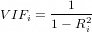# Multicollinearity for newbies

Multicollinearity simply occurs when independent variables in the dataset are correlated. Well, that’s putting it rather simply. The truth is that it’s a nuanced concept, and can have a serious impact on your dataset if not dealt with at the right moment.

As a bad analogy, It always reminds me of that one terrible party crasher that claims to know someone important at the party, but just ruins the party’s outcome, making everyone wish they hadn’t come.

So how do we get rid of him/it?

Not so fast. You see, many data science newbies like myself have succumbed to the phenomenon of collinearity. Because like an unwanted guest at the dinner table, it can bluff us, by overstating it’s importance.

Your job as a host is to ask yourself the following questions. What is it really? How much damage can it cause? What is meant by high collinearity versus perfect collinearity? what can be done to overcome it, and in the rare instance that it cannot be evicted, should we run the model anyway?

So let’s re-cap. When two or more independent variables are perfectly collinear, it means that that one of them can be expressed as a function of the other. For example if there’s perfect collinear relationship between X₁ and X₂ it may hold true that X₁=2X₂ or that X₁=5-(1/3)X₂ or any other relationship. This poses a big problem for your model, because it would be impossible to calculate OLS estimates of the parameters, and the system of normal equations will contain two or more equations that are not independent. This is perfect collinearity.

High collinearity, on the other hand is easier to deal with, if we can diagnose it correctly. Here the OLS estimate coefficients are still unbiased. If your principal aim is a predictive model, then in many cases we can simply drop the errant independent variable with the higher P-value significance. High collinearity can also be sometimes corrected by extending the size of the sample data, transforming the functional relationship or by just dropping one of the variables. I must admit, I’ve used the latter way more than I probably should. (Oh, the shame)

Detecting multicollinearity is a simple affair with metrics like variance inflation factor, that quantify the severity, and is measured by the formulaHere Ri is the coefficient of determination of the ith variable to the rest of the independent variables.

In the case of a high VIF factor, we simply drop the variable with the higher integer value, and carry on. But dropping variables won’t really help us when the significance level is high and the variables are highly collinear to boot. (This is the party crasher who’s also your dad’s friend from school)

Illustrating with an example, suppose we have a classic economic Cobb Douglas production function, where

Where Q represents output, K, L and A represent capital, labour and productivity or technology. If we represented this relationship linearly, we would get glaring R² values of ~0.96 or higher, indicating a strong collinearity between K and L. But at the same time both L and K are highly statistically significant, and dropping either leads to a biased slope estimate for the retained variable because economics postulates that both labour and capital have to be included in the production function.

So how can we overcome the situation? Well, if we know that the relationship of labour and capital indicates constant returns to scale, a phenomena by which If production increases by the some proportional change, then all factors of production are also changing by the same proportion, and we can represent constant returns, or that α + β =1, then we can rewrite the function as,

lnQ=α LnL+β LnK +Ln A + μ

And now simplifying, taking the natural log on both sides, and rewriting in terms of α we can say,

lnQ=Ln A+ α LnL+(1-α) LnK, or simplifying to

lnQ-lnK =ln A+ α (lnL — lnK)

and representing (lnQ-lnK) as lnQ* and (lnL — lnK) as lnL*

and regressing lnQ* on lnL*, we get a linear relationship on the lines of

lnQ* =ln A+ α(lnK*)

What happened here? we have transformed the functional relationship, then formulated the linear equation and factored the target variable into the model itself. This lends a more stable outcome R² to the process.

So the next time that unwanted crasher shows up in the wrong context, don’t shut him out, put him in a clown suit, and make him the life of the party.

## More from Merrill Sequeira

https://github.com/consumer-git/DataProjects